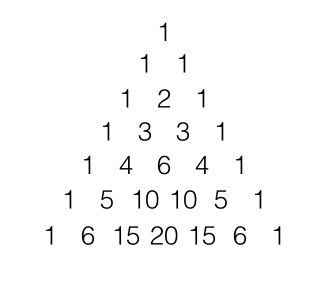## Pascal's Triangle

2017 May 6

Whew! I need a break from iterated functions.

Prove that the sum of the entries of the nth row of Pascal's Triangle is $2^n$. Prove that the alternating sum of the entries of the nth row of Pascal's Triangle is $0$. Find a formula for

The mathematical phenomenon known as "Pascal's Triangle" recently caught my eye. Pascal's Triangle is a triangular arrangement of numbers stretching on to infinity with each row of numbers containing one number more than the previous row. Furthermore, each number is the sum of the two numbers above it, with the topmost number a one. Here are the first $7$ rows of pascal's triangle:This mathematical entity has some interesting properties. For example, if we denote the combinations formula for "n choose r" by

and we call the topmost row of Pascal's triangle row 0 and the first entry in each row the 0th entry in that row, then the rth item in the nth row is given by $_nC_r$. This can be proven easily, because since each entry in the triangle is the sum of the entries above it, and since $_0C_0$ is trivially $1$, also the topmost entry of the pyramid, we need only prove that this summing property holds for $_nC_r$, or that

Here is a quick algebraic proof of that identity. We start with the expression and expand it out using our formula:

We can now see that this is clearly equal to $_nC_r$. This can be useful in proving some conjectures about patterns found in Pascal's triangle. For example, observe what happens when one alternates addition and subtraction for each entry in some row (other than the 0th row):

We can obviously conjecture that this will always be $0$, but how can we prove it? Well, we can do so using what we now know about the connection between Pascal's Triangle and the combinations formula. Such an alternation of addition and subtraction will take the form

Using our identity $nC_r={n-1}C_{r-1}+_{n-1}C_r$, we can expand this out to get

and we can rearrange this to get

This is, of course, a telescoping sum, and we are left only with

Of cource, any $_kC_k$ or $_kC_0$ is equal to $1$, so we now have

Those two terms will be of opposite signs, and we get $0$ as a final result, proving our conjecture.

Now notice what happens when you sum the entries of each row:

We can make yet another conjecture: that the sum of the entries of the nth row is equal to $2^n$. We can prove this using a similar technique, but first we must modify our identity a little bit. Right now we know that

and so

Simply by subtracting, we get that

This identity will prove useful in this proof. Let us denote the sum of the entries of the nth row by $S(n)$. Then

By substitution from our identitu, we get

We can rearrange this to get or

This is where the trick comes in. Notice that the sum

Is the same as $S(n)$, and the sum

Is the same as $S(n+1)$, so we have now that

and this crunches down to

Great! Now we've defined the sum in terms of itself recursively, and since $S(0)=1$, we can say that $S(n)=2^n$, since the sum of each row is twice the sum of the previous row.

Alright, there's one more interesting problem to do, and it doesn't at first seem like it will have anything to do with the combinations operator. The problem comes from @Ryan on Math StackExchange. The problem is to find a general formula for the iterated summation

For this problem, it isn't hard to make a conjecture. Here are the first couple iterates:

It is easy to see just by observing the pattern that

Here is the proof that I posted on Math Stackexchange:

I have already shown that the conjecture holds for the first two, so that part is out of the way. Our conjecture is that

Assume that this statement is true for some $n_k$. Then we must prove that its truth for some $n_k$ implies its truth for $n_{k+1}$, or that if the above is true, then

Must also be true. This may at first seem like a scary problem to attack until we remember that we assumed that

was true, allowing us to substitute and instead have the task of proving

Which is less intimidating. First one must notice that the quantity

is equal to

and we can substitute this into our equation to get

We can now notice that

is the same as

and so now we have

Now we can attack the sum

Which expands out to form

Now we can use the identity of the combinations formula

to expand out our infinite sum:

We can see now that this is a telescoping sum that condenses down to

When we substitute this into our equation, we get this:

Which is a truth statement. This proves that if our statement is true for some $k$, then is must be true for $k+1$, and since it is true for $k=1$, it is true for all natural numbers $k$. QED.

That was sure a pain to type up.

Anyways, the combination formula's interesting identity seems useful in turning normally difficult summations into telescoping sums.